# Double Deep Q-Networks

Today’s post is about Double Deep Q-Networks. Double Deep Q-Networks is an important enhancement of the standard Deep Q-Networks that I explained in my previous post. We will first see what is the problem with the DQN and how we encounter these problems using the Double DQN. After that, we will implement the Double DQN from scratch, where I will explain each step of the implementation.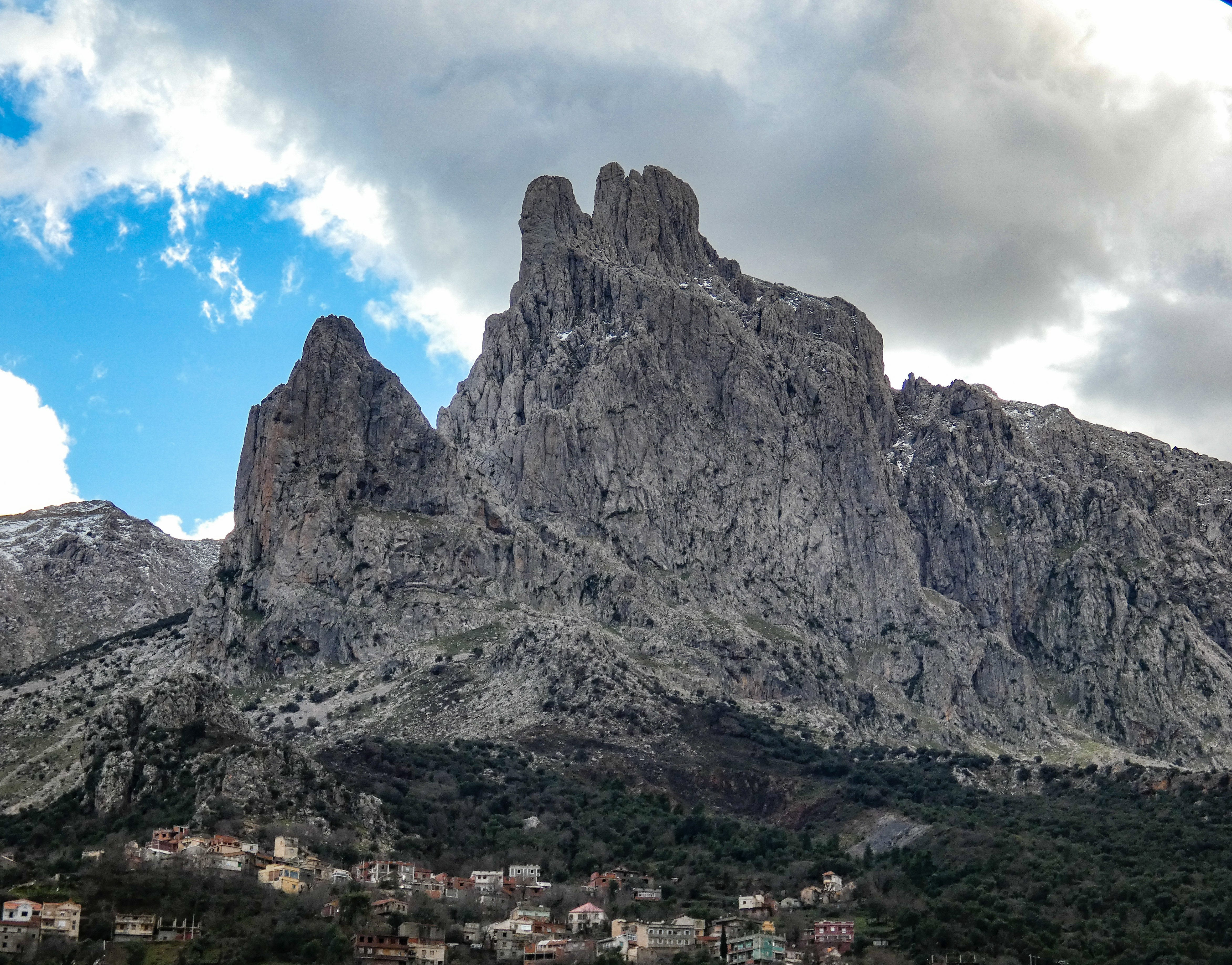Main du juif mountain in Algeria. Taken by me

Let’s recall an important definitions and formulas that we need to master before going deeper.

The goal of the agent is to find a policy that satisfies the Bellman optimality equation for each state s and action a.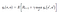Bellman optimality equation

This equation tells us that under the optimal policy, the Q-value of the action a and the state s is equal to the Q-target which is the immediate reward plus the maximum discounted Q-value that we can obtain with the next state s’.

To approach the optimal policy, we use a Deep Neural Network (DNN). The DNN computes the Q-values of different actions given a state. Thus, the first layer of DNN has the same size as the state and the last layer the number of actions that the agent can take.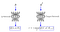from https://towardsdatascience.com/deep-double-q-learning-7fca410b193a

To update the DNN parameters, we store all the experiences of the agent (s, a, r, s’ done), where s is the current state, a the action taken by the agent, r the reward, s’ the next state and done is a boolean telling the agent whether the episode ends or not. Then, after each step, we select randomly a batch of experiences to update the DNN using the Bellman equation.

Both the Q-values and the Q-targets are computed using the same DNN. The DNN’s goal is to reduce the lack between each Q-value and its Q-target by updating its parameters. And here is all the problem. When we update the DNN parameters, we make the Q-value closer to the Q-target but the Q-target is changed and moves in the same direction than the Q-value since we use the same DNN to compute both the Q-value and the Q-target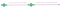By me

This makes the training phase of the agent unstable, so the agent takes more time to converge and leads to bad performances.

To overcome this problem, we use the Double Deep Q-Networks algorithm. The idea is quite simple: Instead of using one DNN to compute both the Q-values and the Q-targets, we use two DNNs. The first one computes the Q-values and the second one the Q-targets. After a certain number of experiences, we update the parameters of the Q-target neural network by copying those of the Q-value neural network. We understand that these two DNNs have the same architecture.

Implementation

Let’s implement the Double DQN algorithm from scratch using Keras and TensorFlow. I will explain each step of the implementation and finish with an example where an agent learns to play CartPole game.

Well, let’s start by importing some libraries we need

Then, we will create a class representing our agent and define some methods that we will use. The code is documented, so you can easily understand all the process:

Now we can start learning the CartPole game using our agent. In CartPole, we have a pole standing on a cart which can move. The goal of the agent is to keep the pole up by applying some force on tit every time step. When the pole is less than 15° from the vertical, the agent receives a reward of 1. An episode is ended when the pole is more than 15° far from the vertical or when the cart position exceeds 2.4 units from the centre.

We instantiate the environment and set some parameters as the number of episodes

After 500 iterations, the learning is done. We can show how the agent behaves using the following code:

I got the following result. The result will not be the same for another episode.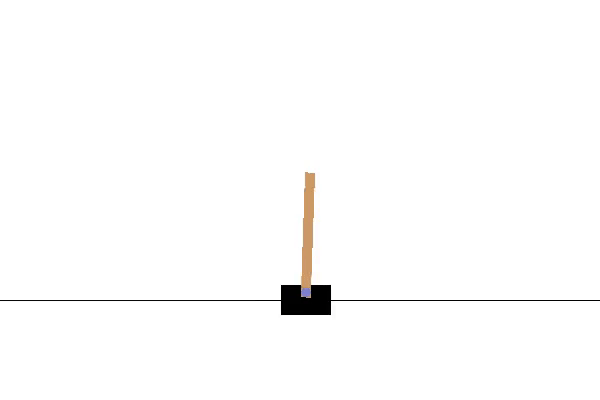My agent playing CartPole.

The DDQN allows the agent to converge quickly and to be more accurate. In this post, we only explained the DDQN algorithm. I will try to prepare another post where I will compare the performances of a standard DQN agent against a DDQN one. I hope you enjoyed this post. Please let me know if you have any questions or comments.

Thank you!

5th year computer science and statistics engineering student at Polytech Lille, I am fascinated by machine learning and Graph Neural Networks.

## More from Amrani Amine

5th year computer science and statistics engineering student at Polytech Lille, I am fascinated by machine learning and Graph Neural Networks.